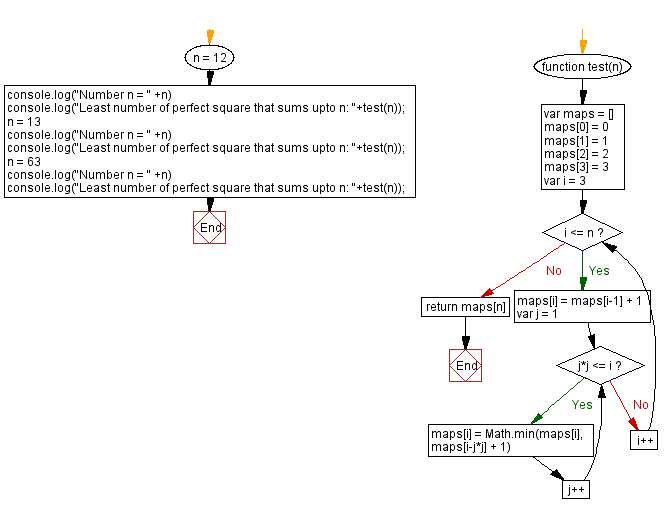# JavaScript: Least number of perfect square that sums upto n

## JavaScript Math: Exercise-106 with Solution

Write a JavaScript program that accepts an integer (n) as input and calculates the lowest number of exact square numbers that sum to n.

In mathematics, a square number or perfect square is an integer that is the square of an integer; in other words, it is the product of some integer with itself. For example, 9 is a square number, since it equals 32 and can be written as 3 × 3.

Example:
Input: 12
Output: 3
Explanation: 12 = 32 + 12 + 12
Input: 63
Output: 4
Explanation: 63 = 72 + 32 + 22 + 12.

Test Data:
(12) -> 3
(13) -> 2
(63) -> 4

Sample Solution:

HTML Code:

``````<!DOCTYPE html>
<html>
<meta charset="utf-8">
<title>JavaScript program to Least number of perfect square that sums upto n</title>
<body>

</body>
</html>
```
```

JavaScript Code:

``````function test(n) {
var maps = []
maps = 0
maps = 1
maps = 2
maps = 3

for (var i = 3; i <= n; i++) {
maps[i] = maps[i-1] + 1
for (var j = 1; j*j <= i; j++) {
maps[i] = Math.min(maps[i], maps[i-j*j] + 1)
}
}
return maps[n]
}

n = 12
console.log("Number n = " +n)
console.log("Least number of perfect square that sums upto n: "+test(n));
n = 13
console.log("Number n = " +n)
console.log("Least number of perfect square that sums upto n: "+test(n));
n = 63
console.log("Number n = " +n)
console.log("Least number of perfect square that sums upto n: "+test(n));
```
```

Sample Output:

```Number n = 12
Least number of perfect square that sums upto n: 3
Number n = 13
Least number of perfect square that sums upto n: 2
Number n = 63
Least number of perfect square that sums upto n: 4
```

Flowchart:Live Demo:

See the Pen javascript-math-exercise-106 by w3resource (@w3resource) on CodePen.

Improve this sample solution and post your code through Disqus

Previous: Conversion of integers to English words.
Next: Power of three.

What is the difficulty level of this exercise?

Test your Programming skills with w3resource's quiz.

﻿